# Abstract

We propose two policies to select the route for an adaptive-alternative routing algorithm for all-optical networks. We use the NrPSR to find the Nr routes with lower cost for a given source-destination pair according to a cost function expanded in a power series (PSR) in which the coefficients are determined by a Particle Swarm Optimizer (PSO). The selected route to attend to the call request can be chosen among the Nr found routes depending on the adopted policy. In the first proposal, named NrPSR(OSNR JE), we select the route that presents the lower OSNR that attends the Quality of Transmission requirements. In the second approach, named NrPSR(OSNR MAX), we select the route that presents the higher OSNR. In our simulations we considered some physical layer effects, such as: ASE noise generation, Optical Amplifier gain and ASE saturation and OXC crosstalk. We compared the performance of our proposed policies to others previous proposed policies for the NrPSR and other well known algorithms described in the literature. NrPSR(OSNR JE) outperformed all other routing algorithms.

Optical Networking; Routing and Wavelength Assignment; Wavelength Division Multiplexing; Particle Swarm Optimization

IPolytechnic School of Pernambuco, University of Pernambuco, Recife, PE, Brazil 50.720-001 E-mail: carmelofilho@ieee.org

IIDepartment of Electronics and Systems, Federal University of Pernambuco, Recife, PE, Brazil 50.740-550 Email: jfmf@ufpe.br

ABSTRACT

We propose two policies to select the route for an adaptive-alternative routing algorithm for all-optical networks. We use the NrPSR to find the Nr routes with lower cost for a given source-destination pair according to a cost function expanded in a power series (PSR) in which the coefficients are determined by a Particle Swarm Optimizer (PSO). The selected route to attend to the call request can be chosen among the Nr found routes depending on the adopted policy. In the first proposal, named NrPSR(OSNRJE), we select the route that presents the lower OSNR that attends the Quality of Transmission requirements. In the second approach, named NrPSR(OSNRMAX), we select the route that presents the higher OSNR. In our simulations we considered some physical layer effects, such as: ASE noise generation, Optical Amplifier gain and ASE saturation and OXC crosstalk. We compared the performance of our proposed policies to others previous proposed policies for the NrPSR and other well known algorithms described in the literature. NrPSR(OSNRJE) outperformed all other routing algorithms.

Index Terms: Optical Networking, Routing and Wavelength Assignment, Wavelength Division Multiplexing, Particle Swarm Optimization.

I. INTRODUCTION

Optical Communications Systems have been considered as an efficient solution to provide high transmission rates with relative low cost. Optical networks are networks that use optical links connected by optical cross-connectors (OXC) . They can be classified in three types: opaque, translucent and transparent. In opaque optical networks, the signal is regenerated in all intermediate nodes of the lightpath for a given source-destination pair. In transparent optical networks (also known as all-optical networks), the signal is transmitted entirely in the optical domain, without suffering Optical-Electrical-Optical regenerations. In translucent optical networks, some of the nodes have regenerators and the signal can be regenerated in some of them according to a predefined policy. In this paper, we are considering all-optical networks, since they present a lower cost and the constraints imposed by the physical layer are more severe. It occurs due to the noise accumulation and distortion along the transparent lightpath  .

There are two main challenges in all-optical networks: (i) to design an efficient routing and wavelength assignment (RWA) algorithm, which has a direct influence in the network performance, and (ii) to obtain an acceptable optical signal-to-noise ratio (OSNR) at the destination node for each lightpath . These requirements are necessary for providing quality of service (QoS) .

The RWA problem consists in to find a route and to assign a wavelength for each connection request. The RWA process can be classified in two types: static or dynamic . In the static case, the call requests are known in advance and the RWA operations can be performed in an off-line manner. The main objective is to minimize the number of wavelengths needed to establish the call requests for a given physical topology. In the dynamic case, the target is to minimize the blocking probability, while optimizing the availability of resources. In general, the solution for the dynamic RWA problem is simplified by decoupling the problem into two separate sub-problems: the routing (R) and the wavelength assignment (WA) . The performance of a dynamic RWA algorithm can be assessed in terms of the blocking probability (BP), which assesses the chance of failure in the implementation of a given network request. It can occur due to the lack of resources or inadequate quality of transmission (QoT) of the lightpath .

Several solutions can be found in the literature for solving the routing problem in optical networks. In general, they are classified in three types: fixed routing, fixed-alternative routing and adaptive routing. The fixed routing algorithms always choose the same route for a given source-destination pair. The fixed-alternative routing algorithm chooses a route from an ordered list of fixed routes depending on a predefined policy. The adaptive routing algorithms consider the current network state to determine the routes .

Some examples of routing algorithms proposed in previous works are: Shortest Path (SP) , Minimum Hop (MH) and Least Resistance Weight (LRW) . In the SP algorithm, the route is defined based on the minimum total physical link length. The MH algorithm finds the physical route with the minor number of optical links. The LRW algorithm finds the routes based on the wavelength availability aiming to distribute the load over the entire network. One must observe that the LRW algorithm uses the current information of the network i.e. adaptive algorithm, whereas the two formers are fixed routing algorithms.

Another relevant aspect of the RWA is the wavelength assignment algorithm (WA). There are some well known WA algorithms, such as: First-Fit (FF), Random Pick (RP), Least Used (LU), Must Used (MU) and Distributed Relative Capacity Loss (DRCL) . In this paper, we are considering the MU algorithm. The MU algorithm selects the most used wavelength in the entire network and it keeps the spare capacity of less-used wavelengths. We selected the MU algorithm to be deployed in this paper since it is simple and presents a good performance for WDM networks.

Recently, we have proposed an adaptive-alternative routing algorithm for optical networks, called NrPSR . The NrPSR algorithm finds the Nr routes that present the lower cost, which are evaluated based on a cost function expanded in a power series . Then, it chooses one of them based on a pre-defined policy. In this paper, we propose two alternative policies based on the Optical Signal-to-Noise Ratio (OSNR). In the first proposal, named NrPSR(OSNRJE), we select the route that presents the lower OSNR that attends the Quality of Transmission requirements. In the second approach, named NrPSR(OSNRMAX), we select the route that presents the higher OSNR.

The rest of the paper is organized as follows. In section II, we present some related works and a description of the NrPSR algorithm. In section III, we describe our proposals, the NrPSR(OSNRJE) and NrPSR(OSNRMAX). In the section IV, we detail the simulation setup and the parameters used in the simulations. In section V, we analyze the performance of our proposals and compare them to other policies for the NrPSR algorithm and some other well know routing algorithms. In section VI, we give our conclusions.

II. RELATED WORK

In this section, we review some contributions that are closely related to this work. Xavier et al.  proposed an adaptive-alternative routing algorithm to solve the RWA joint problem in optical networks, called NrPSR. The NrPSR pseudocode is shown in Algorithm 1. The NrPSR uses the Yen's algorithm in order to find the Nr routes with lower cost. Each route is evaluated using the PSR cost function considering the link length and the link availability as the input variables. The NrPSR can aggregate the benefits of an adaptive RWA by considering the physical layer effects and can include information about the wavelength assignment policy since it evaluates dynamically some possible routes between the source-destination nodes.

Yen  proposed an efficient algorithm to find the Nr paths with lower cost from a source node to a destination node. This algorithm is more efficient then other approaches because its computational upper bound increases linearly with the Nr value.

After finding the set of candidate routes to attend to a connection request, the algorithm NrPSR evaluates each route and selects one of the Nr routes according to a predefined policy. Xavier et al.  proposes the analysis of three policies: Capacity Loss (CL), Minimum Wavelength Matching Factor (MinK) and Maximum Wavelength Matching Factor (MaxK).

Chaves et al. proposed two approaches to solve the routing problem in transparent optical networks: Physical Impairment Aware Weight Function (PIAWF)  and Power Series Routing (PSR) . Both algorithms use an adaptive link weight function that considers two simple network information to consider the physical layer impairments. The PSR proposes an expansion in a power series to evaluate the cost of a link during the calculations of the routes. The first step is to choose the input variables for the cost function. They used the link availability and the link length.

The availability Xi,j (between the nodes i and j) is defined as  :where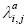andare, respectively, the number of active wavelengths and the total number of wavelengths in the link from node i to node j.

The normalized physical link length yi,j is defined as  :in which di,j is the link length between the nodes i and j, and dmax is the maximum link length in the network.

The second step is to describe the cost function in terms of a power series in agreement with the chosen parameters , as depicted in equation (3).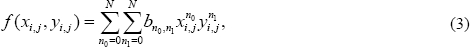whereis the cost function associated to the link between the nodes i and j. N is an integer that represents the number of terms used in the power series expansion.

The third step is to use a global optimizer in order to find thecoefficients for the power series. The PSR uses the Particle Swarm Optimizer (PSO) . The coefficients are calculated in an offline manner, i.e. before the network operation. We also chose to use the PSO algorithm because it can tackle optimization problems in hyper-dimensional search spaces with continuous variables.

The PSO is a population based algorithm and was proposed by Kennedy and Eberhart in 1995 . Each particle l has a position in the search space, that represents a possible solution for the problem. The positions are updated based on the velocities of the particles. And the velocities of the particles depend on the best position obtained by the particle during the search process (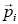) and the best position obtained by the neighborhood of the particle during the search process (). The equations used to update the position and the velocity of the particle are shown in equations (5) and (4), respectively. Algorithm 2 depicts the pseudocode of the PSO algorithm.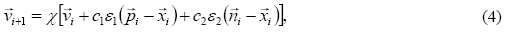in which X is the constriction factor .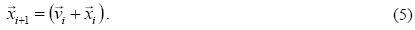Pereira et al. developed a routing algorithm that uses physical layer impairments estimation for the routing decision, named OSNR-R . The goal of this IA-RWA (Impairment Aware Routing and Wavelength Assignment) algorithm is to find the route with higher OSNR (Optical Signal Noise Ratio) for a given wavelength.

III. NRPSR (OSNR) PROPOSALS

In this section, we present two policies for a routing algorithm to solve the RWA joint problem in all-optical networks. In the first, named NrPSR(OSNRJE), we select the route that presents the lower OSNR that attends the QoT requirements. This approach tends to reserve resource for further network requisitions. In the second approach, named NrPSR(OSNRMAX), we select the route that presents the higher OSNR. This one tends to maximize the QoT of the lightpaths. The NrPSR(OSNRJE) pseudocode is shown in Algorithm 3 and the NrPSR(OSNRMAX) pseudocode is shown in Algorithm 4. Both algorithms use the Yen's algorithm to find the Nr routes with lower cost according to the PSR cost function. After finding the set of routes to attend to a call request, the algorithm NrPSR(OSNRJE) evaluates each route and selects the one that presents the lowest OSNR above the QoT criterion and the algorithm NrPSR(OSNRMAX) evaluates each route and selects the one that presents the highest OSNR. After the performance evaluation of the candidate routes, if there is no route to attend to the connection request, the call request is blocked and NrPSR does not test another route.

IV. SIMULATION SETUP

We used the SIMTON simulation tool  to estimate the blocking probability for the RWA algorithms. The SIMTON uses the physical layer model described in . For each network simulation, we run a set of 105 calls randomly generating the source-destination pairs for each connection request. The connection request process is characterized as a Poisson process and the time duration for each established lightpath is characterized by an exponential process. After the selection of a route, if this route is not able to attend to the connection request, either because of lack of resources or inadequate QoT, the connection request is blocked.

We used two topologies in our simulations. The first is the Pacific Bell, shown in Fig. 1, and the second is the Finland, shown in Fig. 2. We assume circuit-switched bidirectional connections in two optical fibers and no wavelength conversion capabilities. The blocking probability is evaluated as the ratio between the number of blocked connection requests and the total number of connection requests. The physical layer impairments considered in this paper are: the ASE (Amplified Spontaneous Emission) and the gain saturation effect in the amplifiers, the PMD (Polarization Mode Dispersion) in the transmission fiber and the crosstalk in the OXC. The optical parameters used in the simulations are shown in Table I, where: Psat is the amplifier output saturation power; OSNRin is the OSNR of the transmitters; Plaser is the output power of the transmitters; OSNRQoS is the minimum OSNR for QoT criterion; B is the transmission bit rate; Bo is the optical fiber bandwidth; W is the number of wavelengths per link; Δf is the channel spacing; λi is the lower wavelength of the grid; λ0 is the zero dispersion wavelength; λ0RD is the zero residual dispersion wavelength; α is the fiber loss coefficient; LMux is the multiplexer loss; LDemux is demultiplexer loss; LSwitch is the optical switch loss; NF is the amplifier noise figure; ε is the switch isolation factor; δ is the maximum pulse broadening; DPMD is the PMD coefficient; SDCF is the compensating fiber slope; and STx is the transmission fiber slope.

The coefficients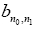for the cost function of NrPSR were optimized for a network load of 60 erlang and the simulation parameters used in PSO are shown in the Table II. In order to evaluate the blocking probability of a given particle, we simulated a set of 105 calls. We used N=5, where N is the number of terms used in the PSR cost function. We used the MU as the wavelength assignment algorithm. The number of pre-selected routes for the NrPSR is Nr=3.

V. SIMULATION RESULTS

In this section, we present the simulation results for the network blocking probability as a function of the traffic load and we perform a load distribution analysis for all investigated RWA algorithms in order to verify the robustness of the algorithm to deal with load variations along the time.

A. Training Phase

The NrPSR requires a training stage to find the optimized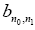coefficients. We executed the PSO to perform the optimization. Fig. 3 and Fig. 4 show the PSO convergence trace for the PSR algorithm and for four different policies used in the NrPSR: (CL), (MaxK), (OSNRMAX) and (OSNRJE), for topologies Pacific Bell and Finland, respectively. One can observe that the algorithms converge very fast and the NrPSR(OSNRJE) algorithm obtained the better performance in terms of blocking probability.

B. Online simulation - comparison with other algorithms from the literature

After the training stage, in which we determined the coefficients, we compared our two proposed policies to two other policies for the NrPSR and four other well known routing algorithms: NrPSR(CL), NrPSR(MaxK), SP, MH, OSNR-R and PSR.

Fig. 5 and Fig. 6 show the blocking probability as a function of the network load for the different routing algorithms for topologies Pacific Bell and Finland, respectively. NrPSR(OSNR) obtained the best performance when compared to the other algorithms. For the topology Pacific Bell, NrPSR(OSNRJE) and NrPSR(OSNRMAX) outperformed all other routing algorithms, mainly for lower network loads. We obtained better results than the OSNR-R routing algorithm. It occurred because our approaches balance the load over the network and also reserves resources for future connection requests. For the topology Finland, NrPSR(OSNRJE) outperformed all other routing algorithms, including our other proposal, NrPSR(OSNRMAX).

In the simulated case shown so far, our proposed algorithm outperformed others routing algorithms. The results shown in Fig. 5 and Fig. 6 also indicate that our proposals keep its performance level even for different loads from the one it was optimized in the training stage. It indicates that our proposals are robust enough to deal with load fluctuations that may occur along the time. Nevertheless, it is also worthy to investigate the robustness of the NrPSR(OSNRJE) and the NrPSR(OSNRMAX) when there are load distribution fluctuations in the network, which results in a traffic pattern different from the one considered during the training stage.

In this section we investigate the performance of the algorithms as a function of the variations of the load distribution. Chaves et al. proposed this analysis in . We can state this verification as follows. Given an uniform traffic matrix T = {ρi,j} (used during the training phase), we produce a variation in this traffic matrix (i.e. T' = {ρ'i,j}), which might simulate the traffic distribution fluctuation along the day. T' is obtained from T by the multiplication of each entry by a random number: {ρ'i,j} = (1 + r) . {ρi,j}, where r is a random number with a uniform distribution in the [-R, R] interval and R is the maximum allowed load variation per source-destination pair. The T' matrix is normalized to keep the same total network load. Hence, R=0 corresponds to the case of uniform traffic, whereas R0 corresponds to a non-uniform traffic. R = 1.0 represents the case of the traffic load of a given source-destination pair may increase or decrease by at most 100% of its traffic in the uniform case.

We selected R=1.0 to perform a statistical analysis of the behavior of the RWA algorithms blocking probabilities. For this purpose, we generated a set of 30 different and independent traffic matrixes. For each one of these matrixes, we obtained the blocking probabilities from the six considered routing algorithms. For PSR and the policies of NrPSR, we used the coefficients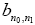values obtained for the uniform traffic. We present the box plot results in Fig. 7 and Fig. 8. The Box stands for the 50% of the obtained data, the whiskers correspond to the 100% of the obtained data, the open symbols stand for the mean value and the horizontal line inside the box represents the median value.

Fig. 7 and Fig. 8 show the Box and Whisker's representations of the statistical analysis of the performance of the algorithms: LRW, OSNR-R, PSR, NrPSR(MaxK), NrPSR(CL), NrPSR(OSNRMAX) and NrPSR(OSNRJE), obtained for a set of 30 different, independent and non-uniform (R = 1) traffic matrixes for the topologies Pacific Bell and Finland, respectively. The NrPSR(OSNRJE) algorithm outperformed all others routing algorithms in both cases.

We used the Wilcoxon non-parametric statistical test  with a significance level of 1%. The results indicated that the results obtained by the NrPSR(OSNRJE) algorithm are statistically significant when compared to the results achieved by the other algorithms. Therefore, at least for these two cases, the NrPSR(OSNRJE) algorithm is the most robust algorithm to deal with load distribution fluctuations.

VI. CONCLUSIONS

In this paper we presented two novel policies to select the lightpath for an adaptive-alternative impairment-aware routing algorithm for all-optical networks, called NrPSR(OSNRJE) and NrPSR(OSNRMAX). We performed simulations in the Pacific Bell and Finland topologies using the Most used WA algorithm. We used a physical impairments evaluation model based on ONSR to consider the following impairments: ASE noise generation, optical amplifier gain and ASE saturations and OXC crosstalk.

We compared our proposals to other well known routing algorithms in two different scenarios and we demonstrated that NrPSR(OSNRJE) outperformed all the others in both scenarios. We also demonstrated that the performance of our proposal is not mitigated if the network traffic load distribution changes, which may occur along the time in real world networks.

Both NrPSR(OSNRJE) and NrPSR(OSNRMAX) algorithms require a training stage to determine the coefficientsbefore the network operation. This characteristic of a priori knowledge of the network behavior reduces the computational time for the routing decision process as compared to noise based online routing calculation approaches.

ACKNOWLEDGMENT

The authors acknowledge the financial support from UPE, UFPE, FACEPE, CNPq and CAPES for scholarships and grant.

For review 7 Dec 2012

Accepted 4 July 2013

•  R. Ramaswami and K. N. Sivarajan, "Optical Networks: A Practical Perspective", 3rd ed., San Diego: Morgan - Kaufmann, 2009.
•  B. Mukherjee, "Optical Communication Networks: Progress and Challenges", Journal of Selected Areas in Communications, v. 18, pp. 1810-1824, n. 10, 2000.
•  M. Gurusamy and C. S. R. Murthy, "WDM Optical Networks: Concepts, Design, and Algorithms", 1st ed. Prentice Hall, 2002.
•  H. A. Pereira, D. A. R. Chaves, C. J. A. Bastos-Filho and J. F. Martins-Filho, "OSNR model to consider physical layer impairments in transparent optical networks", Photonic Network Communication, v. 18, pp. 137-149, 2009.
•  H. Zang, J. P. Jue and B. Mukherjee, "A review of routing and wavelength assignment approaches for wavelength-routed optical WDM networks", Optical Networks Magazine, v.1, pp. 47-60, n. 1, 2000.
•  N. M. Bhide, K. M. Sivalingam and T. Fabry-Asztalos, "Routing mechanisms employing adaptive weight functions for shortest path routing in multi-wavelength optical WDM networks, Photonic Networks Communication, v. 3, pp. 227-236, 2001.
•  B. Wen, R. Shenai and K. Sivalingam, "Routing, Wavelength and time-slot-assignment algorithms for wavelength-routed optical WDM/TDM networks", IEEE/OSA J. Lightware Technology, v. 23, pp. 2598-2609, n. 9, 2005.
•  A. V. S. Xavier, R. C. L. Silva, C. J. A. Bastos-Filho, D. A. R. Chaves and J. F. Martins-Filho, "An Adaptive-Alternative Routing Algorithm for All-Optical Networks", International Microwave and Optoelectronics Conference (IMOC), pp.719-723, 2011.
•  D. A. R. Chaves, D. O. Aguiar, C. J. A. Bastos-Filho and J. F. Martins-Filho, "A Methodology to Design the Link Cost Functions for Impairment Aware Routing Algorithms in Optical Networks", Photonic Network Communications, pp. 1-18, 2011.
•  J. Yen, "Finding the k shortest loopless paths in a network", Management Science, v. 17, pp. 712-716, n. 11, 1971.
•  D. A. R. Chaves, D. O. Aguiar, C. J. A. Bastos-Filho and J. F. Martins-Filho, "Fast and adaptive impairment aware routing and wavelength assignment algorithm optimized by offline simulations", Optical Switching and Networking, v. 7, pp. 127-138, n. 3, 2010.
•  M. Clerc and J. Kennedy, "The particle swarm - explosion, stability, and convergence in a multidimensional complex space", IEEE Transactions on Evolutionary Computation, v. 6, pp. 5873, n. 1, 2002.
•  D. A. R. Chaves, H. A. Pereira, C. J. A. Bastos-Filho and J. F. Martins-Filho, "SIMTON: A Simulator for Transparent Optical Networks", Journal of Communication and Information Systems, v. 25,n. 1, 2010.
•  E. L. Lehmann, "Nonparametrics: Statistical methods based on Ranks", Springer, 2006.
• Comparing OSNR based policies for an adaptive-alternative IA-RWA algorithm applied to all-optical networks
Carmelo J. A. Bastos-FilhoI; Rodrigo C. L. SilvaI; Daniel A. R. ChavesI; André V. S. XavierII; Joaquim F. Martins-FilhoII

# Publication Dates

• Publication in this collection
10 Feb 2014
• Date of issue
Dec 2013